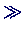## Moments of Inertia: Finding the Eigenvalues of a Matrix

• We look at a rigid body with moments of inertia 1, 3, 5 (in suitable units). In a special coordinate system it is described by the diagonal matrixJ = diag([1 3 5])

J =

1     0     0
0     3     0
0     0     5

• We rotate the system, first by 30 degrees around the x axes, then 45 degrees around the z axes, using the rotation matricesang1 = 30/180*pi

ang1 =

0.5236Q1 = [1 0 0; 0 cos(ang1) sin(ang1) ; 0 -sin(ang1) cos(ang1)]

Q1 =

1.0000         0         0
0    0.8660    0.5000
0   -0.5000    0.8660ang2 = 45/180*pi

ang2 =

0.7854Q2 = [cos(ang2) sin(ang2) 0; -sin(ang2) cos(ang2) 0; 0 0 1]

Q2 =

0.7071    0.7071         0
-0.7071    0.7071         0
0         0    1.0000

• This leads to the following matrix for the moment of inertia in the rotated system:Jrot = Q2*(Q1*J*Q1')*Q2'

Jrot =

2.2500    1.2500    0.6124
1.2500    2.2500    0.6124
0.6124    0.6124    4.5000

• The principal moments of inertia are given by the eigenvalues of Jrot:eig(Jrot)

ans =

1
3
5

• which of course was expected.Peter Junglas 8.3.2000